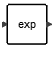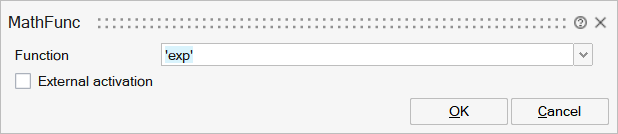# MathFunc

This block applies a function to each entry of its input matrix to produce the corresponding entry of the output matrix. The function can be selected in a set of available functions by the choice of a block parameter.MathOperations

## Description

The MathFunc block implements a set of functions applied to each entry of the input to produce the corresponding output. The function to use is specifed as a block parameter chosen in a list of available functions.

The input datatype should be either double or complex.

This block provides these functions:

exp: exponential function

log: natural logarithm function

10^u: power of ten function

log10: logarithm function with base 10

|u|: absolute value function function

u^2: square function

sqrt: square root function

1/u: inverse function

conj: complex conjugate function

|u|^2: square of absolute value function (norm-2 function)

log2: logarith function with base 2

erf: the error function (also called as Gauss error function) is a sigmoid shape function used mainly in statistics and probability, and partial differential equation. erf is defined aserfc:The complementary error function, denoted erfc, is defined as2^u: poser of two function

round: rounding function

floor: rounding function to smaller integer

ceil: rounding function to larger integer

fix: returns nearest rounded integers toward zero,i.e, y=sign(x).*floor(abs(x)). For positive values behaves like the Floor function and for negative values behaves like Ceil function.

## ParametersNameLabelDescriptionData TypeValid Values

func

Function

String

'exp'
'log'
'log2'
'log10'
'10^u'
'2^u'
'|u|'
'u^2'
'|u|^2'
'sqrt'
'1/u'
'conj'
'erf'
'erfc'
'round'
'floor'
'ceil'
'fix'

externalActivation

External activation

Number

0
1

## Ports

NameTypeDescriptionIO TypeNumber

Port 1

explicit

output

1

Port 2

explicit

input

1

Port 3

activation

input

externalActivation